# 最全INDEX函数教程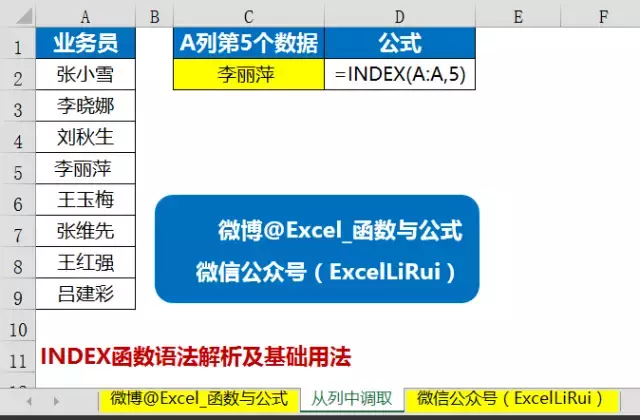C单元格输入以下公式。

=INDEX(A:A,5)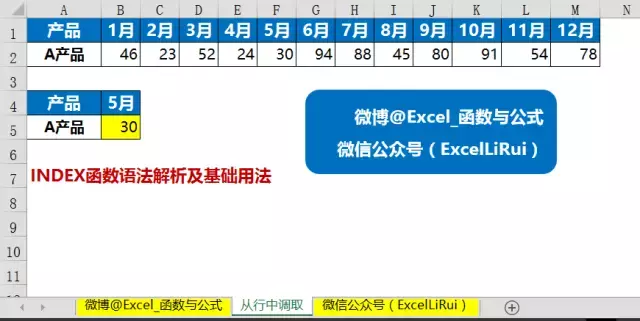B单元格输入以下公式。

=INDEX(2:2:,6)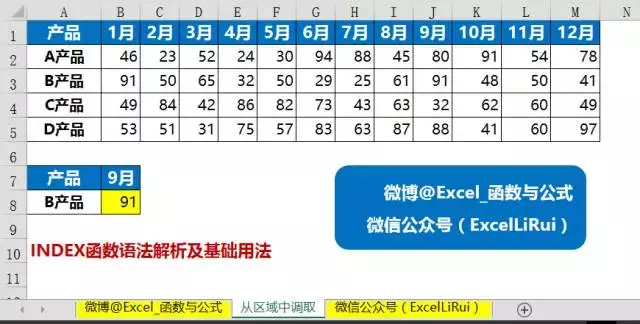B单元格输入以下公式。

=INDEX(B2:M5,2,9)

2、INDEX函数隔行取值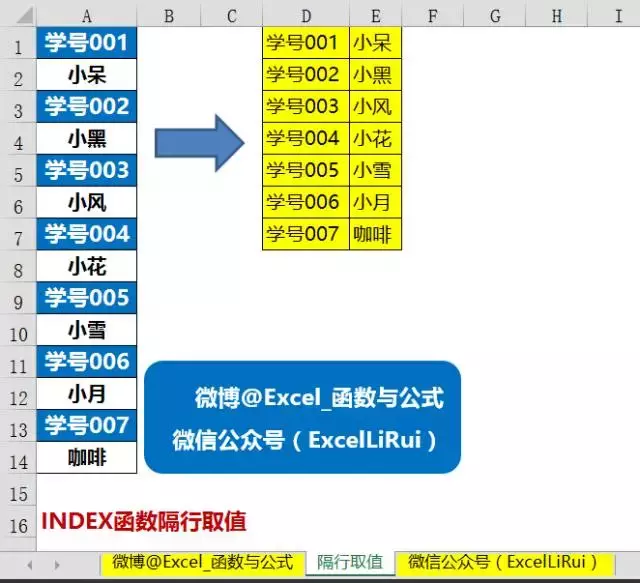=INDEX(\$A:\$A,COLUMN(A1)+(ROW(A1)-1)*2)&""

3、INDEX函数隔列取值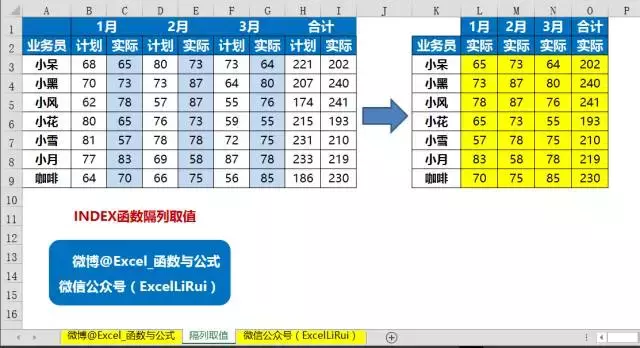=INDEX(\$B3:\$I3,COLUMN(A1)*2)

4、INDEX函数按条件调取整行数据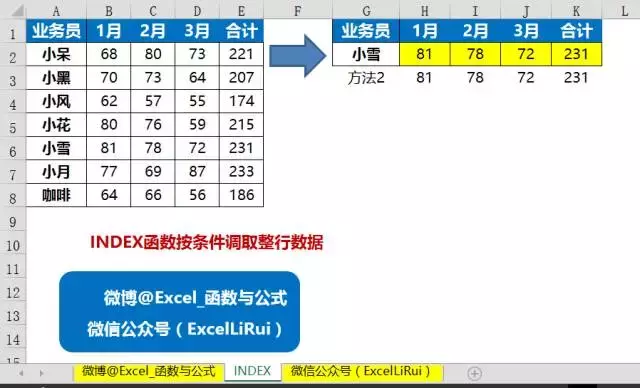=INDEX(\$B\$2:\$E\$8,MATCH(\$G\$2,\$A\$2:\$A\$8,),COLUMN(A1))

5、INDEX函数按条件调取整列数据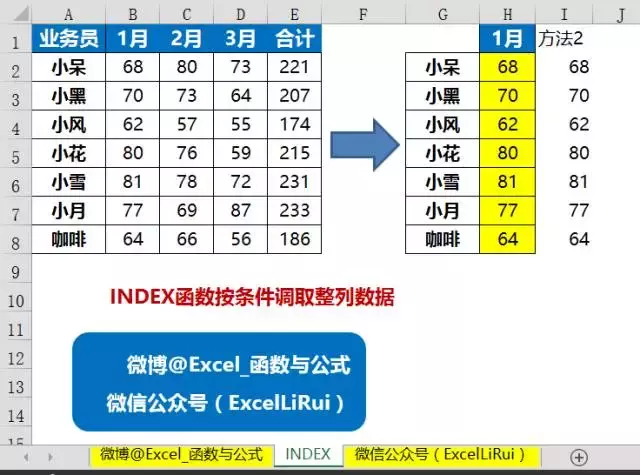=INDEX(\$B\$2:\$E\$8,ROW(A1),MATCH(\$H\$1,\$B\$1:\$E\$1,))

6、INDEX函数二维条件交叉查询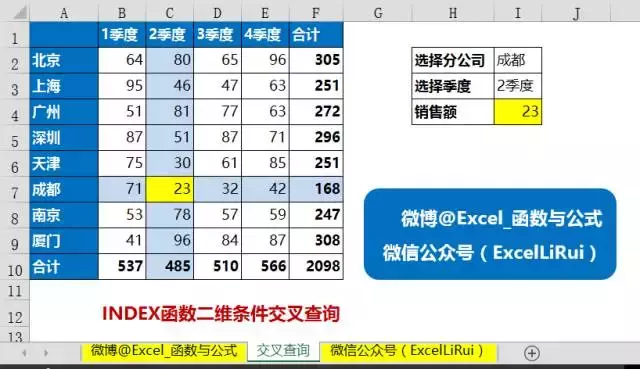I4单元格输入以下公式。

=INDEX(B2:F10,MATCH(I2,A2:A9,),MATCH(I3,B1:F1,))

7、INDEX函数拆分工资表打印工资条

excel函数应用技巧的最大魅力在于实战中体现。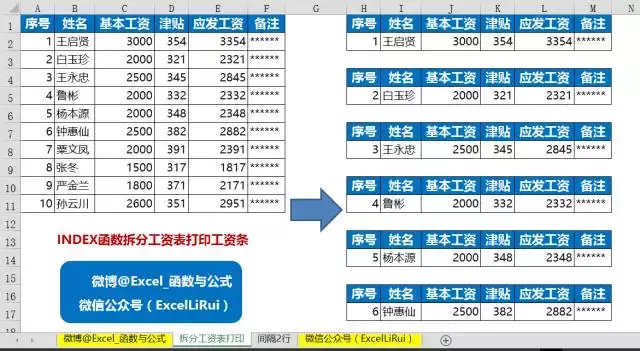=CHOOSE(MOD(ROW(A1),3)+1,"",INDEX(A:A,1),INDEX(A:A,1+INT(ROW(A2)/3)))

8、INDEX函数工资条合并工资表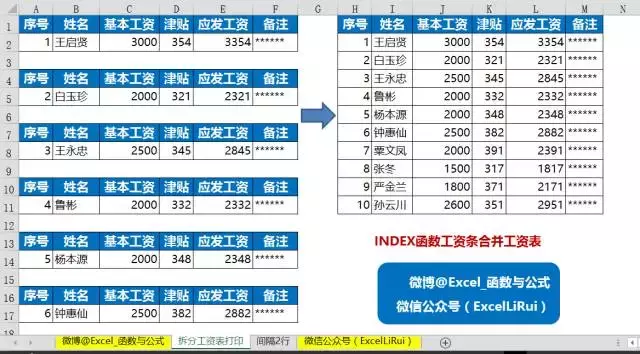=INDEX(A:A,IF(ROW(A1)=1,1,2+(ROW(A1)-2)*3))

9、INDEX函数返回查找到的多个值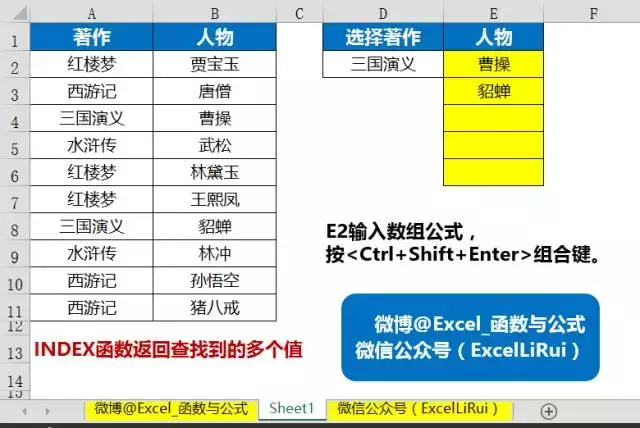=INDEX(B:B,SMALL(IF(A\$2:A\$11=D\$2,ROW(\$2:\$11),4^8),ROW(A1)))&""

10、INDEX函数将单列数据转换为多列排布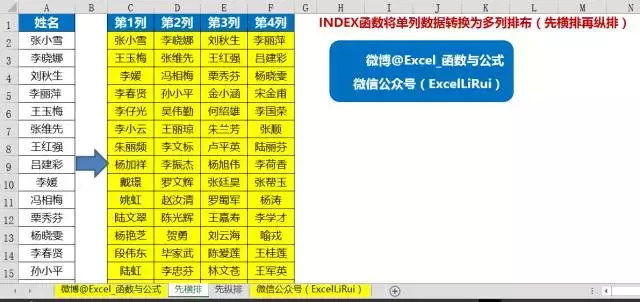=INDEX(\$A:\$A,1+COLUMN(A1)+(ROW(A1)-1)*4)&""